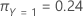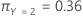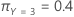# Methods and formulas for misclassification in CART® Classification

Select the method or formula of your choice.

The misclassification table is not present when the splitting method is class probability.

## Count

When there are no weights, the counts and the sample sizes are the same.

## Weighted count

In the weighted case, the weighted count is the sum of the weights for a category. Weighted counts round to the nearest whole number. Use the unrounded weights to calculate percentages and rates. Consider the following simple example:
Response level Predicted level Weight
Yes Yes 0.1
Yes Yes 0.2
Yes No 0.3
Yes No 0.4
No No 0.5
No No 0.6
No Yes 0.7
No Yes 0.8
This table provides the following statistics:
Actual class Weighted count Misclassed Predicted Class = No Percent correct
Yes 0.1 + 0.2 + 0.3 + 0.4 = 1 0.1 + 0.2 = 0.3 ≈ 0 0.3 + 0.4 = 0.7 ≈ 1 (0.3 / 1.0) ×100 = 30%
No 0.5 + 0.6 + 0.7 + 0.8 = 2.6 ≈ 3 0.7 + 0.8 = 1.5 ≈ 2 0.5 + 0.6 = 1.1 ≈ 1 1.1 / 2.6) × 100 = 42.31%
All 1 + 2.6 = 3.6 ≈ 4 0.3 + 1.5 = 1.8 ≈ 2 0.7 + 1.1 = 1.8 ≈ 2 (0.3 + 1.1) / 3.6 × 100 = 38.89%

## % Error

In the weighted case, use weighted counts in place of counts.

## Cost

The calculation of cost depends on whether the response variable is binary or multinomial.

Cost = (% Error × Input misclassification cost for class) / 100

### Binary response variable

The following equation gives the cost for the event class:

The following equation gives the cost for the non-event class:

The following equation gives the overall cost for all classes:

### Multinomial response variable

For the multinomial case, the equation extends the formula for the binary response variable to account for all the possible types of misclassifications. For example, for a multinomial response with k classes, the misclassification cost for Y = 1 uses the following equation:

The following equation gives the overall cost for the multinomial case:

For example, consider a response variable with 3 classes and the following misclassification costs:

 Predicted Class Actual class 1 2 3 1 0.0 4.1 3.2 2 5.6 0.0 1.1 3 0.4 0.9 0.0

Then, consider that the following table gives the error percentages:

 Predicted Class Actual class 1 2 3 1 N/A 1% 0.5% 2 1.4% N/A 2.1% 3 5% 1.2% N/A

Finally, consider that the classes of the response variable have the following prior probabilities:

•••The following equations give the costs associated with the misclassification for each class in the response variable:

The following equation gives the overall cost: